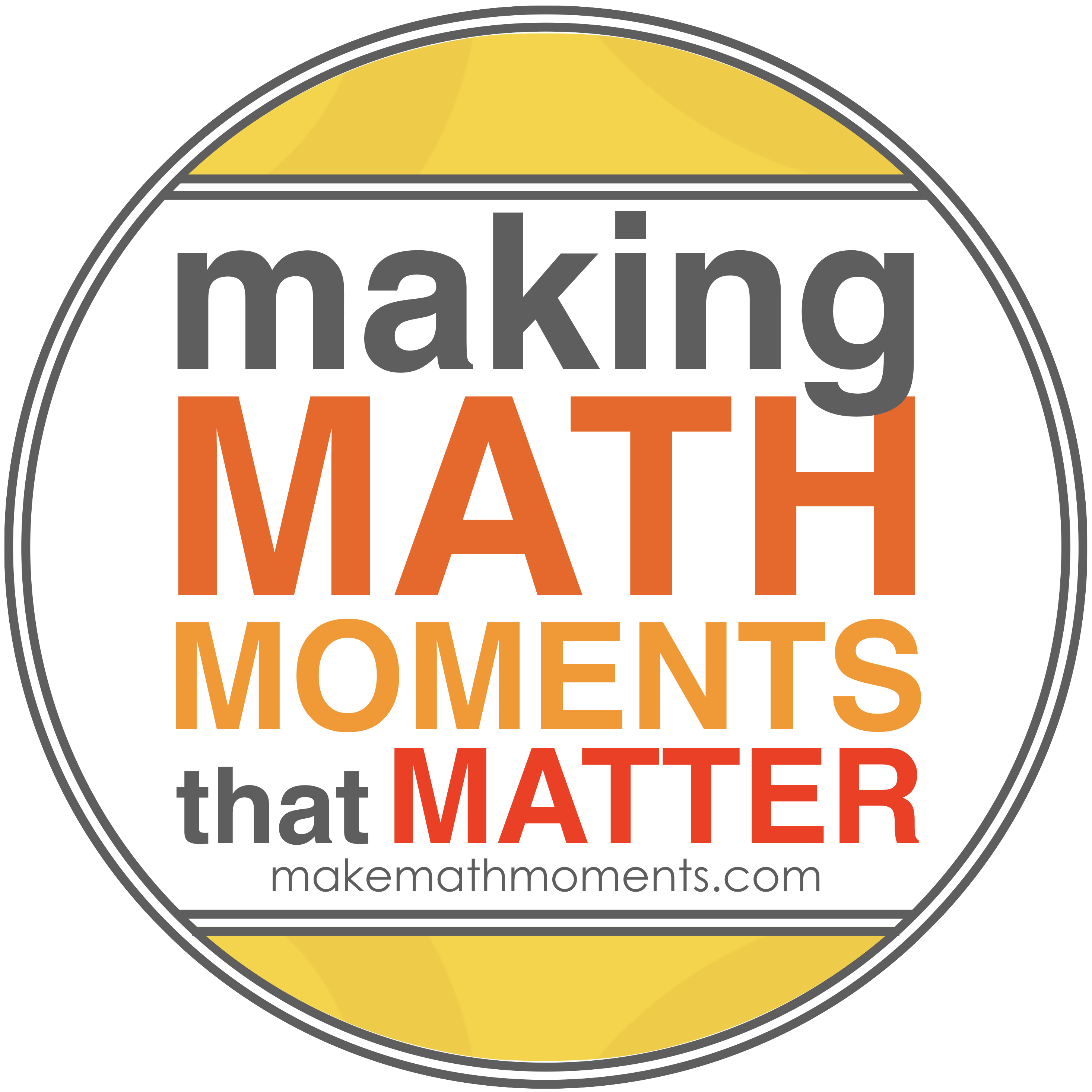# MPM1D

 COURSE NAME:  Principles of Mathematics, Grade 9 COURSE CODE:  MPM 1D1 MINISTRY GUIDELINE:  The Ontario Curriculum, Grades 9 and 10,                                                Mathematics, 2006 (Revised) LEVEL OF DIFFICULTY:  Academic CREDIT VALUE:  1 PREREQUISITE:  none TEXTBOOK(s): Principles of Mathematics 9 McGraw-Hill Ryerson Value : \$75

Course Description:

This course enables students to develop an understanding of mathematical concepts related to

algebra, analytic geometry, and measurement and geometry through investigation, the effective

use of technology, and abstract reasoning. Students will investigate relationships, which they

will then generalize as equations of lines, and will determine the connections between different

representations of a linear relation. They will also explore relationships that emerge from the

measurement of three-dimensional figures and two-dimensional shapes. Students will reason

mathematically and communicate their thinking as they solve multi-step problems.

Overall Learning Expectations:

By the end of this course, students will:

• demonstrate an understanding of the exponent rules of multiplication and division, and

apply them to simplify expressions;

• manipulate numerical and polynomial expressions, and solve first-degree equations.
• apply data-management techniques to investigate relationships between two variables;
• demonstrate an understanding of the characteristics of a linear relation;
• connect various representations of a linear relation.
• determine the relationship between the form of an equation and the shape of its graph with

respect to linearity and non-linearity;

• determine, through investigation, the properties of the slope and y-intercept of a linear

relation;

• solve problems involving linear relations.
• determine, through investigation, the optimal values of various measurements;
• solve problems involving the measurements of two-dimensional shapes and the surface areas

and volumes of three-dimensional figures;

• verify, through investigation facilitated by dynamic geometry software, geometric properties

and relationships involving two-dimensional shapes, and apply the results to solving

problems.

Evaluation:

Term Work                                                                                        70%

Knowledge / Understanding                                    30 %

Inquiry / Thinking                                                20 %

Communication                                                20 %

Application / Making Connections                        30 %

Summative assessment activities during the course will be comprised of a variety of methods and strategies.  (eg.  Assignments, projects, tests, journals, performances, conferences, etc.)

Final Evaluation                                                                              30%

The final evaluation will consist culminating activities which include, but are not limited to:

• a final examination, written during the examination schedule (15%)
• the Grade 9 EQAO Assessment of Mathematics. (15%)

Classroom Rules:

Students are expected to follow these rules so that mathematics education can be successful.

a) BE ON TIME

b) COME PREPARED FOR CLASS

c) PARTICIPATE IN CLASS – Keep good notes while the lesson is being taught, pay

attention in class, ask questions and answer any questions the teacher asks, if possible.

d) RESPECT THE RIGHTS OF OTHERS – Work quietly and co-operate so as not to

disturb other students who are trying to learn.

e) CATCH UP ON ANY WORK MISSED – When returning from an absence, catch up

on missed work as quickly as possible.

f) COMPLETE ANY HOMEWORK ASSIGNED – If work is not completed in class,

complete it before the next class so that you may benefit from the discussions based on

the homework.

g) REMAIN IN YOUR DESK until the teacher dismisses the class.

h) FOLLOW ESTABLISHED SCHOOL ROUTINES AND BEHAVIOUR CODES – see

student handbook.

Learning Materials Required:

• Textbook: Book Number: __________
• Notebook – 3 ring binder with lined and graph paper
• Pencil, eraser, ruler
• Scientific calculator (TI 83/84 preferred but not mandatory)
• Student Handbook

## 2 thoughts on “MPM1D”

1.I am a Math teacher in Ontario and have found your website very resourceful. I would like to share some resources with you, and would like your email to send you some files that I have created. If you are interested, please send me an email so that I can forward you some files.

2.3dfdnbzngjwc423gf3xcbnf2bmyuwcdbmyuzcrzcdnf2bmkzcsbhkwcsnhz## CONTACT ME